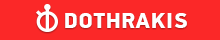GMAT Question of the Day - Daily to your Mailbox; hard ones only

 It is currently 26 Jun 2019, 03:37GMAT Club Daily Prep

Thank you for using the timer - this advanced tool can estimate your performance and suggest more practice questions. We have subscribed you to Daily Prep Questions via email.

Customized
for You

we will pick new questions that match your level based on your Timer History

Track

every week, we’ll send you an estimated GMAT score based on your performance

Practice
Pays

we will pick new questions that match your level based on your Timer History

Not interested in getting valuable practice questions and articles delivered to your email? No problem, unsubscribe here.r and n are integers such that r≥4 and n≥4. If (r-3)(n-3)<5, what is

Author Message
TAGS:

Hide Tags

Math ExpertV
Joined: 02 Sep 2009
Posts: 55802
r and n are integers such that r≥4 and n≥4. If (r-3)(n-3)<5, what is  [#permalink]

Show Tags00:00

Difficulty:25% (medium)

Question Stats:73% (01:36) correct27% (01:26) wrongbased on 50 sessions

HideShow timer Statisticsr and n are integers such that r≥4 and n≥4. If (r-3)(n-3)<5, what is the greatest possible value of n ?

A. 4
B. 5
C. 6
D. 7
E. 8

_________________
CEOP
Joined: 18 Aug 2017
Posts: 3936
Location: India
Concentration: Sustainability, Marketing
GPA: 4
WE: Marketing (Energy and Utilities)
Re: r and n are integers such that r≥4 and n≥4. If (r-3)(n-3)<5, what is  [#permalink]

Show Tags

Bunuel wrote:
r and n are integers such that r≥4 and n≥4. If (r-3)(n-3)<5, what is the greatest possible value of n ?

A. 4
B. 5
C. 6
D. 7
E. 8

r has to be mini so r =4 and n can be at max be 7 so that ((r-3)(n-3)<5 IMO D
_________________
If you liked my solution then please give Kudos. Kudos encourage active discussions.
VPP
Joined: 31 Oct 2013
Posts: 1376
Concentration: Accounting, Finance
GPA: 3.68
WE: Analyst (Accounting)
Re: r and n are integers such that r≥4 and n≥4. If (r-3)(n-3)<5, what is  [#permalink]

Show Tags

Bunuel wrote:
r and n are integers such that r≥4 and n≥4. If (r-3)(n-3)<5, what is the greatest possible value of n ?

A. 4
B. 5
C. 6
D. 7
E. 8

Given

r≥4
n≥4

(r-3)(n-3)<5

i must prefer back solving here.

minimum value of r must be 4. Therefore , n must be 7. The product has to be lower than 5.

(r-3)(n-3)<5

(4-3) (7-3) <5.

Thus , n is maximum 7.Re: r and n are integers such that r≥4 and n≥4. If (r-3)(n-3)<5, what is   [#permalink] 29 Nov 2018, 06:08
Display posts from previous: Sort by

r and n are integers such that r≥4 and n≥4. If (r-3)(n-3)<5, what is